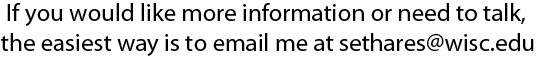Some Useful Computer ProgramsHere are some computer programs useful in understanding and implementing dissonance-based tunings and timbres. Since I usually program in the language MATLAB, most of these are MATLAB programs, although the dissonance calculating program is also available in Microsoft's version of BASIC. Morgan Thunder has also translated the dissonance measuring program into C, and updated by Prof. Frink while Mauricio Rodriguez has translated it into LISP -- thanks Morgan, Prof. Frink, and Mauricio!

To calculate the dissonance of a sound with a given spectrum, use the following program, which should be placed in your MATLAB folder and called dissmeasure.m
The logical structure and the meaning of the variables are discussed in the section How to Calculate Dissonance Curves of the paper Relating Tuning and Timbre, which is available on-line for your browsing pleasure.

 `function d=dissmeasure(fvec,amp)` `%` `% given a set of partials in fvec, ` `% with amplitudes in amp,` `% this routine calculates the dissonance` `%` Dstar=0.24; S1=0.0207; S2=18.96; C1=5; C2=-5; A1=-3.51; A2=-5.75; firstpass=1; N=length(fvec); [fvec,ind]=sort(fvec); ams=amp(ind); D=0; for i=2:N Fmin=fvec(1:N-i+1); S=Dstar./(S1*Fmin+S2); Fdif=fvec(i:N)-fvec(1:N-i+1); a=min(ams(i:N),ams(1:N-i+1)); Dnew=a.*(C1*exp(A1*S.*Fdif)+C2*exp(A2*S.*Fdif)); D=D+Dnew*ones(size(Dnew))'; end d=D;

Dissonance curves are made by calling the above function repeatedly. For instance, the following code calculates and plots the dissonance curve for a harmonic spectrum with 7 partials of equal amplitude, as shown in figure 3 of the paper "Relating Tuning and Timbre"

 freq=500*[1 2 3 4 5 6 7]; amp=ones(size(freq)); range=2.3; inc=0.01; diss=; % % call function dissmeasure for each interval % for alpha=1+inc:inc:range, f=[freq alpha*freq]; a=[amp, amp]; d=dissmeasure(f, a); diss=[diss d]; end plot(1:inc:range,diss)

A pair of useful MATLAB programs converts from musical ratios into cents, and back again. These are

 function cents=rat2cent(ratios) % % convert ratios to cents % cents=1200/log10(2)*log10(ratio); function ratios=cent2rat(cents) % % convert cents to ratios % ratio=10.^((log10(2)/1200)*cents);

which should be named rat2cent.m and cent2rat.m and placed in your MATLAB folder. As usual in MATLAB, the input variables cents and ratios can be scalars or vectors.

For those without access to MATLAB, here is a version of the dissonance measuring program written in BASIC

 DIM freq(10),amp(10),g(10),diss(1500)   ' Variables you must set: ' n=number of frequencies in timbre f ' freq(i) = frequency value of ith partial ' amp(i) = amplitude of ith partial   ' loop through all intervals from startint to endint   dstar=.24: s1=.0207: s2=18.96: c1=5.0: c2=-5.0 a1=-3.51: a2=-5.75: index=-1 PRINT " Interval Dissonance" startint=1: endint=2.3: inc=.01 FOR alpha=startint TO endint STEP inc index=index+1: d=0 FOR k=1 TO n g(k)=alpha*freq(k) NEXT k ' calculate dissonance between f and alpha*f FOR i=1 TO n FOR j=1 TO n IF g(j)To get to my homepage, click here.